Lists

Creation and calculation of lists

Calculation with Lists

The RedCrab calculator can create and calculate multi-dimensional data array. The following section describes, how to handle one-dimensional lists.

To generate a list, a sequence of numbers or variables are written in square brackets and separated by commas.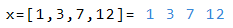The creation of a series from 2 to 8 shows the following example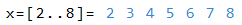A series expands automatically in steps of + /- 1.
Other step sizes can be generated by multiplying or dividing the array.
In addition, step size can be specified in the array definition.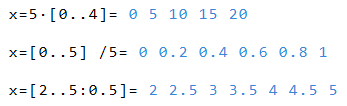Series, values ​​and variables can be combined.Mathematical formulas must be written in parentheses. In the definition of a series or step sizes, no formulas may be used.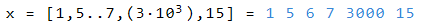A fraction bar is regarded as a value and must not be written in parentheses.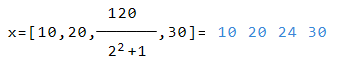Fields are treated as normal values ​​in calculations and they can be used with all operators and functions.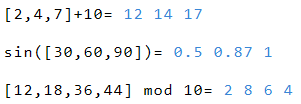The next example shows a result list, which contains four different values ​​of four different arguments of f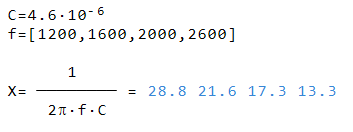Access to individual components of a list are possible via the index